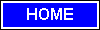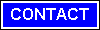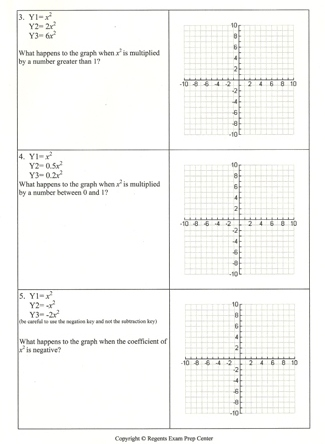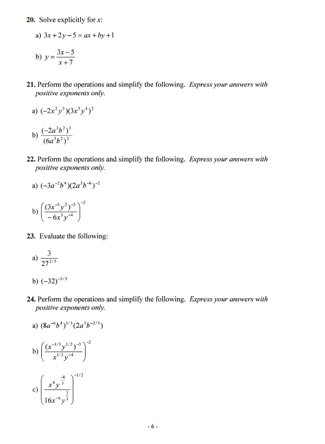M A T H   T U T O R I N G
ONE-ON-ONE
TEST PREPARATION## 917.392.7004    email: mathtutor255@yahoo.com

### Solving Equations and inequalities, fractions , decimals, Graphs and Functions (Graphing points, lines, finding the slope of the line, Functions, Parallel and perpendicular lines ) Systems of Equations and Inequalities (Solving systems of equations graphically, system of inequalities) Polynomials and Factoring (Combining like terms, Multiplication of polynomials, Sums and differences of cubes , Factoring by grouping ) Fractional Expressions (Combining like terms, Multiplication , Division, Lowest Common Multiple , Addition and subtraction , Complex fractions, Variation , direct and indirect) Powers and Roots(Square roots , Cube roots Multiplication and simplification Roots and exponents , Radical equations Complex Numbers (Imaginary numbers , Graphing complex numbers ) Quadratic Equations(Solving quadratic equations , Quadratic formula , Quadratic Functions, Symmetry , Transformations , Parabolas Coordinate Geometry (Distance and midpoint formulas, Circles , Ellipses , Hyperbolas , Exponential and Logarithmic Functions, Inverse functions , Exponential functions , Logarithmic functions, Probability (Permutations , Combinations Matrices (Addition and subtraction, Multiplication Polynomials and Factoring Fractional Expressions (Sums and differences of cubessitemap